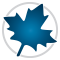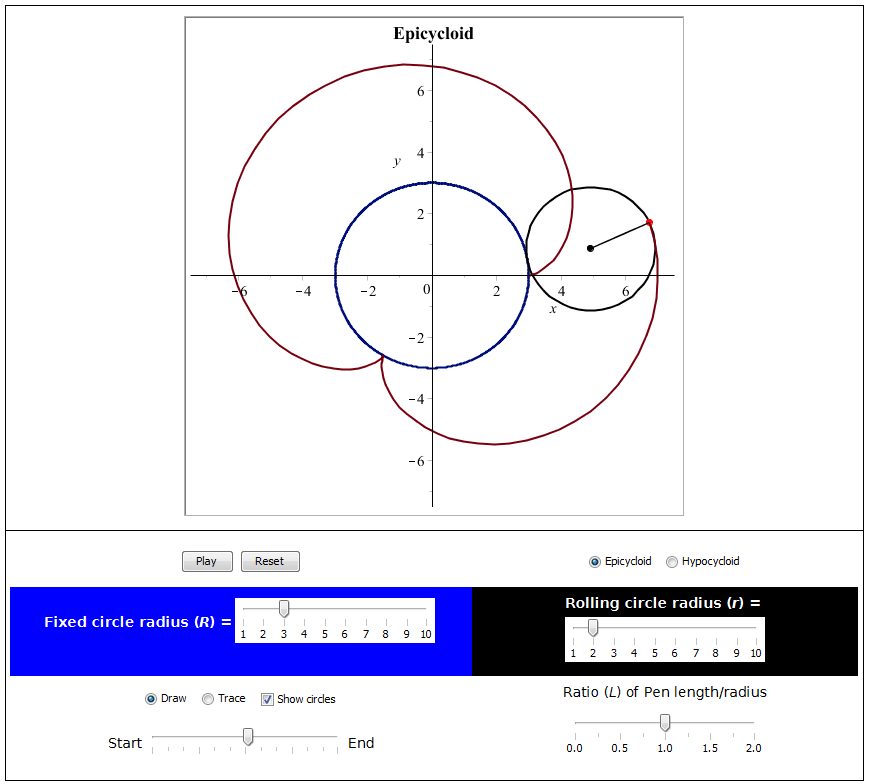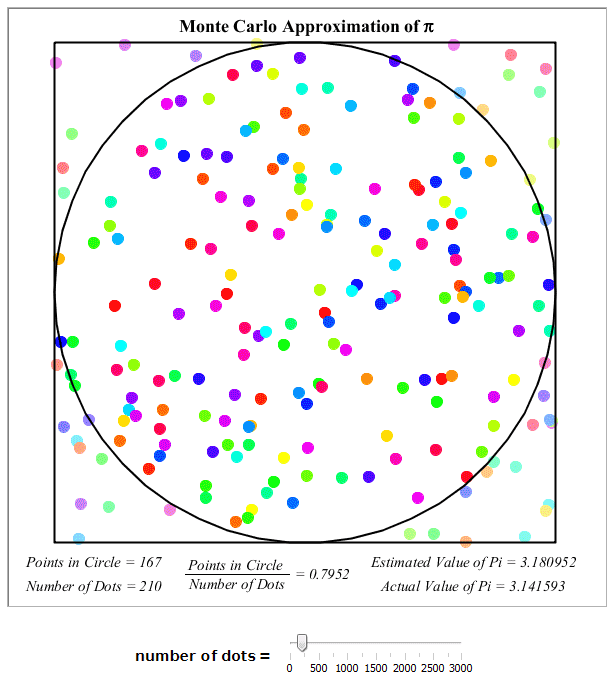Math Apps - Maple Features - Maplesoft# Maple

Math AppsDemonstrations in Maple use interactive features and visualization to explore mathematical concepts. You can manipulate the inputs and instantly see the effects. The demonstrations can be incorporated into any learning environment, such as classroom demonstrations or independent studying.

• Over 400 Math Apps illustrate a broad set of fundamental mathematical concepts
• Point-and-click approach makes it easy to visualize and experiment with mathematical concepts
• Topics include functions and graphing, calculus, statistics, physics, and more
• Maple code used in the demonstrations can be easily viewed, modified, and copied, so it can be used as a starting point for your own work

Example: Drawing the Graph of a Quadratic Function

The following example allows the student to learn how to draw the graph of a quadratic equation (a parabola).

Generate a quadratic function and try to draw it on the graph. Use the radio buttons below the graph to graph different elements in different colors. When you are finished, click the corresponding check boxes to see how accurate you were.

Example: Epicycloids and Hypocycloids

This next app allows the student to user sliders, buttons and checkboxes to adjust the parameters of an epicycloid, hypocycloid, epitrochoid or hypotrochoid, the equations for which are given by:Example: The Monkey and the Coconut
The following classic thought experiment, The Monkey and the Coconut is used to illustrate the effect of the gravitational force on a projectile.

A coconut tree is growing in the middle of a small river. Two monkeys arrive at the bank and notice a small ripe coconut about to fall into the river. In order to get the coconut, the monkeys make a plan: On the count of three, the first monkey will shake the tree, while the other will jump across the river and catch the coconut as it falls, landing on the far bank.

How should the second monkey aim her jump in order to catch the coconut?

Adjust the monkey's initial speed and jump angle so that she catches the coconut.

Note: Air resistance is negligible, and time has been slowed down.

Monte Carlo Approximation of π

This example from statistics shows how to use the probabilistic Monte Carlo method to estimate the value of π:

1. A number of points are selected at random within a square with an inscribed circle
2. The number of points inside the circle divided by the total number of points will result in an approximation of π/4. We multiply by 4 to get the estimate for π.

Adjust the number of points being plotted and see how it affects the approximation of Pi.Prêt(e) pour l'étape suivante ?

*Ce programme d'évaluation n'est proposé actuellement ni aux étudiants ni aux particuliers.

Achetez: